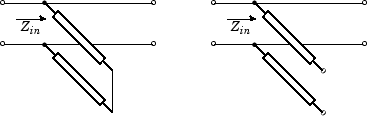# Coaxial Transmission Line

Model coaxial transmission line

•Libraries:
RF Blockset / Equivalent Baseband / Transmission Lines

## Description

The Coaxial Transmission Line block uses S-parameters to model its frequency behavior. A cross-section of the Coaxial Transmission Line is shown in the following figure. Its physical characteristics include the radius of the inner conductor a and the radius of the outer conductor b.## Parameters

expand all

### Main

Radius of the outer conductor of the coaxial transmission line, specified as a scalar in meters.

Radius of the inner conductor of the coaxial transmission line, specified as a scalar in meters.

Relative permeability of the dielectric, expressed as the ratio of the permeability of the dielectric to permeability in free space μ0, specified as a real scalar.

Relative permittivity of the dielectric, expressed as the ratio of the permittivity of the dielectric to permittivity in free space ε0, specified as a real scalar.

Loss tangent of the dielectric, specified as a nonnegative scalar.

Conductivity of the conductor, specified as a positive scalar in Siemens/meters.

Physical length of the transmission line, specified as a positive scalar in meters.

The block enables you to model the transmission line as a stub or as a stubless line.

#### Stubless Transmission Line

• `Not a stub`Not a stub

If you model a coaxial transmission line as stubless line, the Coaxial Transmission Line block first calculates the ABCD-parameters at each frequency contained in the modeling frequencies vector. It then uses the `abcd2s` function to convert the ABCD-parameters to S-parameters. For more information, see Stub Mode - Not a Stub.

#### Shunt Transmission Line

• `Shunt`—This parameter provides a two-port network that consists of a stub transmission line that you can terminate with either a short circuit or an open circuit as shown in these diagrams.Zin is the input impedance of the shunt circuit and it is calculated as follows:

for a short circuited transmission line

`${\text{Z}}_{\text{in}}=j{Z}_{0}\mathrm{tan}\gamma l$`

and for the open circuited transmission line

`${\text{Z}}_{\text{in}}=-j{Z}_{0}\mathrm{cot}\gamma l$`

where

• Z0 is the characteristic impedance and

• $\gamma$ is the propagation constant

The ABCD-parameters for the shunt stub are calculated as

`$\begin{array}{c}A=1\\ B=0\\ C=1/{Z}_{in}\\ D=1\end{array}$`

#### Series Transmission Line

• `Series`—This mode parameter provides a two-port network that consists of a series transmission line that you can terminate with either a short circuit or an open circuit as show in these diagrams.Zin is the input impedance of the series circuit. The ABCD-parameters for the series stub are calculated as

`$\begin{array}{c}A=1\\ B={Z}_{in}\\ C=0\\ D=1\end{array}$`

Stub termination for stub modes `Shunt` and `Series`. Choices are `Open` or `Short`

#### Dependencies

To enable this parameter, select `Shunt` or `Series` in Stub mode

### Visualization

Frequency data source, specified as `User-specified`.

Frequency data range, specified as a vector in hertz.

Reference impedance, specified as a nonnegative scalar in ohms.

Type of data plot to visualize using the given data, specified as one of the following:

• `X-Y plane` — Generate a Cartesian plot of the data versus frequency. To create linear, semilog, or log-log plots, set the Y-axis scale and X-axis scale accordingly.

• `Composite data` — Plot the composite data. For more information, see Create Plots Using Equivalent Baseband Library Blocks.

• `Polar plane` — Generate a polar plot of the data. The block plots only the range of data corresponding to the specified frequencies.

• `Z smith chart`, ```Y smith chart```, and `ZY smith chart` — Generate a Smith® chart. The block plots only the range of data corresponding to the specified frequencies.

Type of parameters to plot, specified as one of the following.

 `S11` `S12` `S21` `S22` `GroupDelay` `GammaIn` `GammaOut` `VSWRIn` `VSWROut` `OIP3` `IIP3` `NF` `NFactor` `NTemp` `TF1` `TF2` `TF3` `Gt` `Ga` `Gp` `Gmag` `Gmsg` `GammaMS` `GammaML` `K` `Delta` `Mu` `MuPrime`

Note

Y parameter1 is disabled when you select Plot type to `Composite data`.

Type of parameters to plot, specified as one of the following.

 `S11` `S12` `S21` `S22` `GroupDelay` `GammaIn` `GammaOut` `VSWRIn` `VSWROut` `OIP3` `IIP3` `NF` `NFactor` `NTemp` `TF1` `TF2` `TF3` `Gt` `Ga` `Gp` `Gmag` `Gmsg` `GammaMS` `GammaML` `K` `Delta` `Mu` `MuPrime`

Note

Y parameter2 is disabled when you select Plot type to `Composite data`.

Plot format, specified as one of the following.

Y parameter1Y format1
`S11`, `S12`, `S21`, `S22`, `GammaIn`, `GammaOut`, `TF1`, `TF2`, `TF3`, `GammaMS`, `GammaML`, and `Delta`.`dB`, `Magnitude (decibels)`, `Abs`, `Mag`, `Magnitude (linear)`, `Angle`, `Angle(degrees)`, `Angle(radians)`, `Real`, `Imag`, and `Imaginary`.
`GroupDelay``ns`, `us`, `ms`, `s`, and `ps`.
`VSWRIn` and `VSWROut`.`Magnitude (decibels)` and `None`.
`OIP3` and `IIP3`.`dBm`, `W`, and `mW`.
`NF` `dB` and `Magnitude (decibels)`.
`NFactor`, `K`, `Mu`, and `MuPrime`.`None`
`NTemp``Kelvin`
`Gt`, `Ga`, `Gp`, `Gmag`, and `Gmsg`.`dB`, `Magnitude (decibels)`, and `None`.

#### Dependencies

To enable Y format1, set Plot type to `X-Y plane`.

Plot format, specified as one of the following.

Y parameter2Y format2
`S11`, `S12`, `S21`, `S22`, `GammaIn`, `GammaOut`, `TF1`, `TF2`, `TF3`, `GammaMS`, `GammaML`, and `Delta`.`dB`, `Magnitude (decibels)`, `Abs`, `Mag`, `Magnitude (linear)`, `Angle`, `Angle(degrees)`, `Angle(radians)`, `Real`, `Imag`, and `Imaginary`.
`GroupDelay``ns`, `us`, `ms`, `s`, and `ps`.
`VSWRIn` and `VSWROut`.`Magnitude (decibels)` and `None`.
`OIP3` and `IIP3`.`dBm`, `W`, and `mW`.
`NF` `dB` and `Magnitude (decibels)`.
`NFactor`, `K`, `Mu`, and `MuPrime`.`None`
`NTemp``Kelvin`
`Gt`, `Ga`, `Gp`, `Gmag`, and `Gmsg`.`dB`, `Magnitude (decibels)`, and `None`.

#### Dependencies

To enable Y format2, set Plot type to `X-Y plane`.

Frequency plot, specified as `Freq`.

Frequency plot format, specified as one of the following.

 `Auto` `Hz` `kHz` `MHz` `GHz` `THz`

Y-axis scale, specified as `Linear` or `Log`.

#### Dependencies

To enable this parameter, set Plot type to ```X-Y plane```.

X-axis scale, specified as `Linear` or `Log`.

#### Dependencies

To enable this parameter, set Plot type to ```X-Y plane```.

Plot the specified data using the plot button.